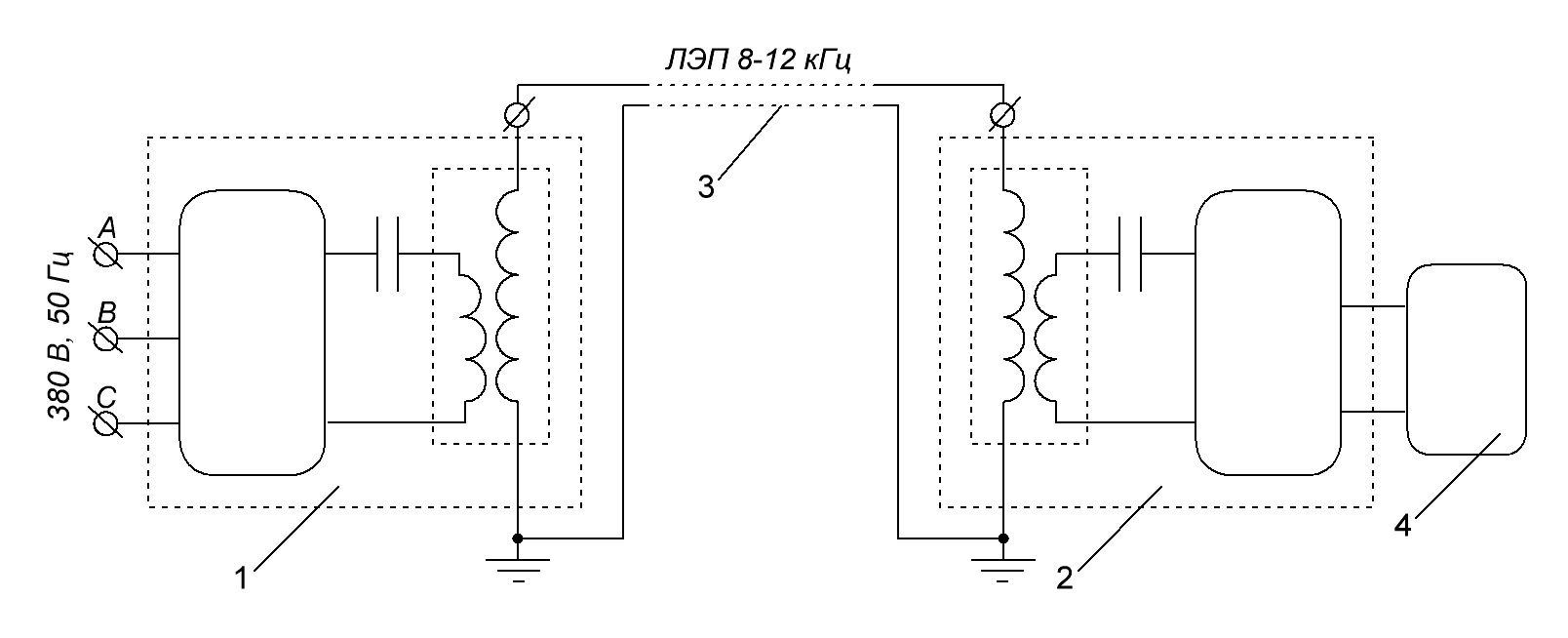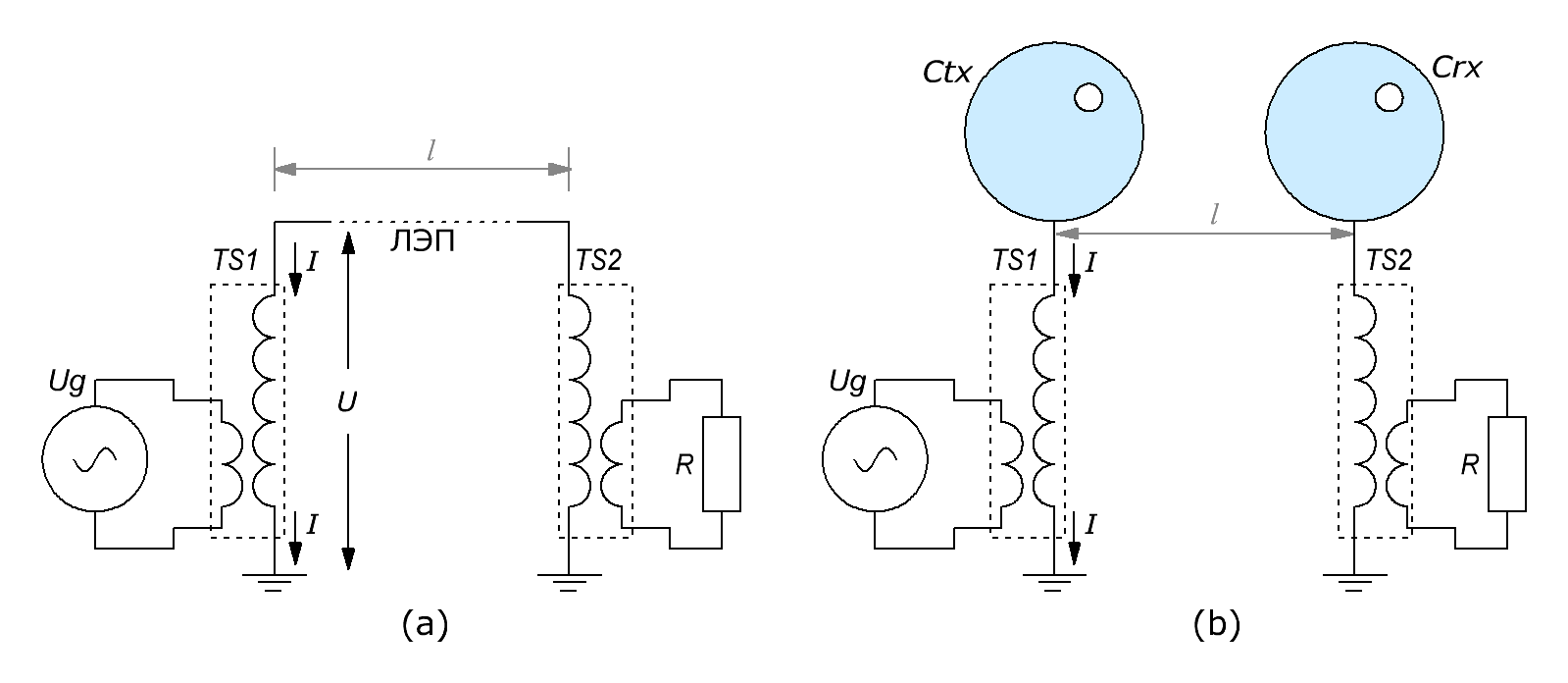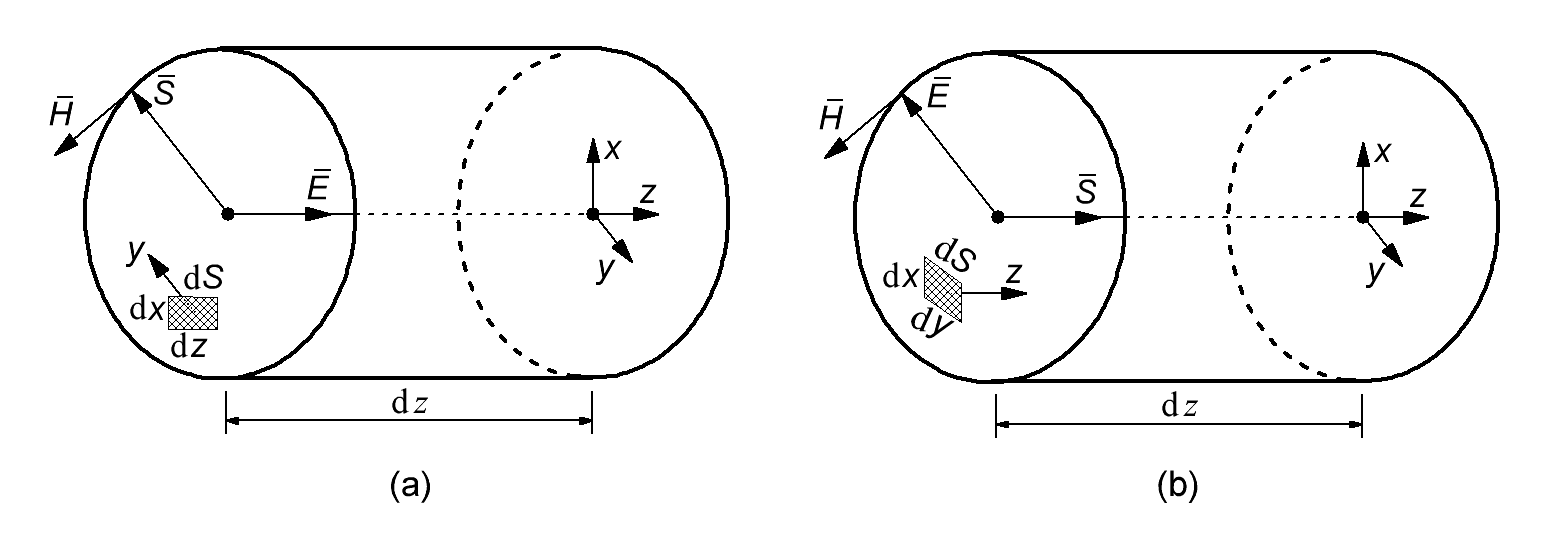Research website of Vyacheslav Gorchilin
2020-12-27
Two kinds of energy in one wire
Authors: Gorchilin V.V., Znamenskiy A.V.
This paper will consider resonant single-wire electrical systems (ROES) and their calculation. This is another example, when at the junction of two branches of physics, unusual and, at first glance, not quite classical results can be obtained. In this case, we are talking about the 'watershed' between electrical engineering and electrodynamics, in which the first one studies electrical processes with frequencies up to 1 kHz, and the second - more than 30 kHz. It is in this frequency range that the conditions are formed under which an ordinary wire begins to acquire some properties of a superconductor, in terms of transmission of electric power through it.
Here we will approach the problem from the most general positions, using for this the law of conservation of electromagnetic energy, covering their entire balance in the conductor. Based on this, we will derive two types of energy, propagating along it and along it. As a result, we will offer a methodology for calculating ROES and give real examples of calculating existing installations. For ease of reading and correct display of formulas, we have divided this material into several pages.
Let's consider a specific version of the scheme proposed in the framework of the research work of one organization.Fig.1. Connection diagram of ROES equipment
The block diagram of the equipment connection is shown in Figure 1. There are presented:
• 1 - block of a prototype resonant transmitting voltage converter;
• 2 - block of a prototype voltage inverter;
• 3 - resonant single-wire power transmission line (PTL) up to 3000 m long (cable RK 75-7-12 or analog);
• 4 is an inverter that converts the output DC voltage of the receiving module (DC = 650 V) into an industrial three-phase AC (AC = 380 V, 50 Hz).
Such a system consists of a transmitting part, a power transmission line (PTL) and a receiving part (Fig. 2a). In the transmitting part, the conduction current of the sinusoidal generator Ug is converted to mixed currents (conductance + displacement), using the TS1 transformer. The power transmission line transfers these currents to the receiving transformer TS2 , which converts the mixed currents back into a conduction current, which is fed to the load R . The second conductor (we will introduce the concept - "conditional", since grounding can be performed either at one point from the side of the EMF source, or not at all) of the power transmission line is the ground, the conductivity of which, including due to the relatively traditional 50 Hz high frequency network (VLW range of 3-30 kHz), will be considered quite high.Fig.2. Single-wire (a) and wireless (b) power lines
Note. The class of resonant power transmission lines also includes a wireless power transmission system, where the capacitive coupling between the solitary capacities Ctx and Crx is used as the upper conductor (Fig. 2b). Such communication is carried out through the atmosphere of the earth. The disadvantage of this type of energy transfer is its omnidirectionality. More point, in terms of the vector of energy propagation, are single-wire transmission lines, which we will further consider.
The principle of operation of a single-wire transmission line
The principle of single-wire transmission of electricity is actually very simple. Suffice it to recall the experience from the electrostatics section of the school physics course. On the teacher's desk were two isolated metal balls, which can also be called solitary containers, an electrophoretic machine and an electroscope. From it, we remember how the teacher charged such a ball C1 from an electric machine q (Fig. 3a). This ball acquired a certain electric charge, which was shown by the nearby electroscope. Then he turned off the electrophoretic machine and connected a charged ball C1 with an uncharged C2 with a jumper wire (Fig. 3b). In this case, part of the charge flowed into the second ball.
From this course it is known that the entire electrostatic charge is located on the surface of metal objects: not inside, not in the near-surface layer, but on the surface. By connecting a charged ball with an uncharged one, a current will flow along the connecting conductor also along its surface! And as we remember, the conduction current flows inside the conductor. This means that in this experiment we received a completely different type of current, which was named - "displacement current".Fig.3. Experiments from the school physics course (a, b) and the simplest single-wire line (c)
Based on this, now it is quite easy to obtain the principle of a single-wire transmission line (Fig. 3c). To do this, you will need an electrostatic charge generator of variable sign qV , the transmission line itself ( Line ) and a device, which will separate the positive and negative charges at the end of this line. Such a device can consist of two diodes, each of which passes only its own charge sign ( VD1 , VD2 ). After it, there can be a classic active load R , which is at the same time a solitary container C2 (the same ball). Now it is enough for us to recharge this capacity using an alternating electrostatic charge from qV to get a current in a single-wire transmission line.
In this section, only the principle of energy transfer itself was shown. Modern ROES have a more complex scheme and structure, which allows working with both open and closed telecommunication lines, transmitting rather large powers over long distances. Further, using the Umov vector, we will estimate how much power can be transmitted in this way.
1. Energy and power balance in power lines
Theoretical substantiation of operating ROES installations requires a special approach, since the transferred power sometimes exceeds by orders of magnitude the maximum possible for the used transmission line conductor. In addition, in some ROES, grounding is not used as a second conductor. The relatively low frequency in the power transmission line does not allow talking about the transfer of power using a transverse electromagnetic wave. Based on the experimental data and works [1-2], we can assume that in these cases the energy is transferred by means of a displacement current and a longitudinal wave, which we will further justify.
It is known from classical electrodynamics that in a conductor the electric field $$\vec E$$ and the magnetic field $$\vec H$$ are located as follows (Fig. 4a). The resulting wind, which is responsible for energy transfer, in this case is the Poynting vector $$\vec S$$, directed perpendicular to the conductor and the first two vectors. As we said earlier, this option is not suitable for explaining the energy transfer by a longitudinal wave. Since the Poynting vector is a special case of the Umov vector, we may well try another combination of vectors. Let us unfold the electric field vector perpendicular to the conductor, then the energy transfer vector $$\vec S$$ will also unfold, but will now be directed along the conductor (Fig. 4b). In this form, the vector of energy transfer fully satisfies the experimental data and our task, and we will work with it further.Fig.4. Directions of fields in the conductor: a - the vector S is perpendicular to the conductor, b - the vector S is directed along the conductor
By the way, the action of the electric field vector, which is directed perpendicular to the conductor, we can notice near high voltage power lines, when the hair begins to electrify, and this is felt by us in the form of a light breeze. Another manifestation is the ionization of air molecules near such power lines, which manifests itself in the form of crackling and the smell of ozone. But let's get back to theory.
J.C. Maxwell introduced the displacement current to balance his equations as a complement to the conduction current. Much later, this current, by indirect methods, was nevertheless discovered [3-6], but there are still no reliable instruments measuring it. In classic cases, for example, in low voltage (up to 1 kV) and medium voltage (3 - 35 kV) power grids, the bias current is relatively small compared to the conduction current, and therefore the task of measuring it has not yet been posed. In Maxwell's equations , the total current appears as the sum of the displacement current and the conduction current. In terms of density, this is expressed as follows: $j _ {\Sigma} = j_D + j \qquad (1.1)$ where: $$j_D$$ is the displacement current density, $$j$$ is the conduction current density.
We will return to this formula later, when we find its constituent parts separately. Now it is necessary to find the flow of energy through the transmission line, take into account the power in the closed circuit, and from here find the total transmitted power. To solve this problem, we use the law of conservation of electromagnetic energy in an elementary volume : ${\partial w \over \partial t} + \mathrm {div} \vec S = 0 \qquad (1.2)$ Here are: $$w$$ - volumetric energy density of the electromagnetic field, $$\vec S$$ - energy flux density (Umov vector).
This idea was expressed by Umov in 1873, and in contrast to Poynting's solution , opens up the possibility of a mathematical description of the longitudinal energy transfer along the power transmission line. This is what we need. But first, let's expand the flow divergence in Cartesian coordinates: $\mathrm {div} \vec S = {\partial S \over \partial x} + {\partial S \over \partial y} + {\partial S \over \partial z}$ Based on the experimental data, we assume that: $${\partial S \over \partial x} = {\partial S \over \partial y} = 0$$, therefore $\mathrm {div} \vec S = {\partial S \over \partial z}$ This expression shows a fundamental difference from the classical Poynting vector, where $${\partial S \over \partial z} = 0$$. Now consider the volumetric energy density, which is found as follows : $w = {\varepsilon \varepsilon_0 E ^ 2 \over 2} + {\mu \mu_0 H ^ 2 \over 2} \qquad (1.3)$ where $$E$$ is the electric field strength, $$H$$ is the magnetic field strength, $$\varepsilon \varepsilon_0$$ - relative and constant dielectric permittivity, $$\mu \mu_0$$ - relative and constant magnetic permittivity.
We will consider the transmission line in the form of a coaxial capacitor, in which the electromagnetic wave propagates only along the z axis (Fig. 5). In the examples, we will see that the resulting solution will apply not only to power lines in the form of a coaxial cable, but also to a line with an open conductor.
Substituting (1.3) into (1.2), taking the derivatives, and taking into account one spatial direction, this equation can now be rewritten in scalar form: ${\partial S \over \partial z} + \varepsilon \varepsilon_0 E {\partial E \over \partial t} + \mu \mu_0 H {\partial H \over \partial t} = 0 \qquad (1.4)$ Since the operating frequency of the ROES is relatively low (much less than the wavelength), then we will further assume that the power transmission line has lumped parameters and length - $$l$$. Then the energy flux density S will be the same along the entire length, just like other parameters in this equation. Let's rewrite it like this: $S + S_E + S_H = 0 \qquad (1.4)$ Where $S_E = \varepsilon \varepsilon_0 E {\partial E \over \partial t} l, \quad S_H = \mu \mu_0 H {\partial H \over \partial t} l \qquad (1.5)$ The following notation was introduced here: $$S_E$$ - electrical component of the energy flow, $$S_H$$ - magnetic component of the energy flow.
From the specific energy flux, we can find the power by integrating it over the cross-sectional area of ??the conductor: $P_S + P_E + P_H = 0 \qquad (1.6)$ Where $P_E = \int \limits_ {s} S_E \, ds, \quad P_H = \int \limits_ {s} S_H \, ds, \quad s = \pi r ^ 2 \qquad (1.7)$ Here: $$P_S$$ is the total power of the energy flow transmitted through the power transmission line by an electromagnetic wave, $$P_E$$ - electrical component of power, $$P_H$$ - magnetic component of power, $$s$$ - coaxial cross-sectional area.
Now, let's find each element of the last three equations separately.

1 2 3 4 5Materials used
1. N.P. Khvorostenko. Longitudinal electromagnetic waves.
2. V.A. Kuligin. A hymn to mathematics or the Augean stables of theoretical physics.
3. Eichenwald A.A. About the magnetic action of bodies moving in an electrostatic field. - M .: Univ. Typ., 1904 .-- 144 p.
4. V.S. Gudymenko, V.I. Piskunov. Experimental verification of the existence of a magnetic field created by the capacitor displacement currents.
5. V.N. Zadorozhny Displacement current and its magnetic field.
6. Simple method for detecting bias current in a capacitor - http://gorchilin.com/articles/experiment/capacitor_bias_current
7. J. Maxwell. Treatise on Electricity and Magnetism. In two volumes. T. II. Moscow: Nauka, 1989.
8. Izmailov S.V. Course of electrodynamics. Textbook for physics and mathematics faculties of pedagogical institutes. - Moscow, State educational and pedagogical publishing house of the Ministry of Education of the RSFSR, 1962. - 439 p., Pp. 14.
9. Korneva M., Kuligin V. A mathematical error that distorted physics.
10. I.I. Talykova-Bushkevich. Physics. 2014. The energy density of an electromagnetic wave. Poynting vector.